# Nothing but Functions - Data Structures

So far, we've used JavaScript to build the lambda calculus versions of boolean logic and numbers. Indeed, it should come as no surprise that we can do calculations with functions. Now, let's do something less intuitive: store data using nothing but functions.

Lambda calculus allows functions to access arguments in higher lexical scopes; this mechanism is called a closure. We already saw this in boolean functions, which take two values and always return a specific one. We can take this idea a little further to produce our first data structure, a fixed-length list of values called a tuple.

In mathematics, an "n-tuple" is an ordered list of n values. For example, a 3-tuple might be `(2, 3, 5)`. We won't be able to modify the values, but we can construct new tuples based on old ones. However, we will be able to give the fields names, and the result behaves much like a `struct` in C or, in most OOP languages, an object with a few read-only properties and no methods.

The trick we use to build these tuples is pretty nifty. Create a function that takes a few values and does nothing with them — that's our data. Then, it returns a function which, when passed an "accessor function", will pass that accessor function all of the values it was storing! Think of the outermost function as the tuple constructor. We can even make helper functions that take a tuple and pass it a function that retrieves a specific value for us. In traditional JavaScript, it might look like this:

```function build_3tuple(a, b, c) { //<-- this function is the tuple constructor
return function(accessor) { //<-- this function is the "tuple" itself; it can see a, b, and c from here
accessor(a, b, c);
};
}
function get_a(tuple) {
return tuple(function(a, b, c){ return a; }); //give the tuple a function that just returns 'a'
}
function get_b(tuple) {
return tuple(function(a, b, c){ return b; });
}
function get_c(tuple) {
return tuple(function(a, b, c){ return c; });
}

var tuple = build_3tuple(2, 3, 5); //construct a tuple
tuple(function(a, b, c) {
// do something with the values in the tuple!
});
var b = get_b(tuple);```

Under our lambda calculus rules, it looks like this:

```var BUILD_3TUPLE = a => b => c => f => f(a)(b)(c);
//                                \-- "tuple" --/
var GET_A = t => t(a => b => c => a); //take all the values, but only return the one we want
var GET_B = t => t(a => b => c => b);
var GET_C = t => t(a => b => c => c);

var tuple = BUILD_3TUPLE(N0)(N1)(N2);
tuple(a => b => c => .....); //do something with the values in the tuple!
var b = GET_B(tuple);```

With these tools, we can finally get back to numbers and build our missing functions. The first function to build is the opposite of the successor function, called the predecessor (or "decrement" in software), which simply finds `n-1`. This function sounds like it should be simple, but consider what it means in terms of lambda calculus numbers. If a "number" is a function that applies some other function that many times, then the "predecessor of a number" is a function that applies some other function one fewer time than it would have normally. You can't un-apply a function, and so we have to be a little more clever.

First, we need a 2-tuple, more commonly called a pair:

```var PAIR   = a => b => f => f(a)(b);  //PAIR(a)(b) constructs a pair which stores "a" and "b" for later
var FIRST  = p => p(a => b => a);     //given a pair "p", get back "a"
var SECOND = p => p(a => b => b);     //given a pair "p", get back "b"

// Then,
var three_and_one = PAIR(N3)(N1);
FIRST(three_and_one)  == N3;
SECOND(three_and_one) == N1;```

Then, we make a peculiar little function everyone calls "phi" (or "shift-and-increment"). This function takes a pair of numbers, `(a, b)`, and produces a new pair containing the second number and the successor of the second number, `(b, b+1)`. In effect, this acts like increment-but-log-previous: the first half of the pair is always the value before the most recent increment. If we start with `(0,0)` and then keep passing it to `phi()`, the next values are `(0,1)`, `(1,2)`, `(2,3)`, `(3,4)`, and so on. If we do this `n` times, then the second half of the pair is `n`, but the first half is the predecessor of `n`! (Or zero if `n` is zero, since we don't have negative numbers.)

Here's what that looks like in code:

```var PHI    = p => PAIR(SECOND(p))(SUCC(SECOND(p)));
//                     \-first-/  \-- second ---/

var PRED   = n => FIRST(n(PHI)(PAIR(N0)(N0)));
//                \---/ get the first half of..
//                      \----/ applying PHI() n times
//                             \----------/ to the pair (0,0)```

Then, subtraction is easy: to find `m - n`, apply `PRED()` `n` times to `m`, that is, starting from `m`, subtract one `n` times.

`var SUB    = m => n => n(PRED)(m);`

Now that we have subtraction, things like comparing numbers or testing whether a number is zero become more useful. The `ISZERO()` function abuses the way number functions behave: every number function will apply the function they are given at least once except zero, which returns the original value. So, it gives the number in question a function that always returns `FALSE`, and asks it to run "always return `FALSE`" on the value `TRUE`. If the function is run even once, the result goes from `TRUE` to `FALSE`.

`var ISZERO = n => n(x => FALSE)(TRUE);`

Finally, we can abuse the unsigned nature of our numbers to construct a less-than-or-equal (`LEQ()`) function: because any negative number becomes zero, if `m` minus `n` is zero, `m` must be less than or equal to `n`:

`var LEQ    = m => n => ISZERO(SUB(m)(n));`

If we improve our original math demonstration code,

```output(["n","succ","plus","mult","pow","pred","sub(2)","iszero","leq(2)"].join("\t")+"\n");
[N0, N1, N2, N3, N4].forEach(function(n){
output([
l2js_uint(n),
l2js_uint(SUCC(n)),
l2js_uint(PLUS(n)(n)),
l2js_uint(MULT(n)(n)),
l2js_uint(POW(n)(n)),
l2js_uint(PRED(n)),
l2js_uint(SUB(n)(N2)),
l2js_bool(ISZERO(n)),
l2js_bool(LEQ(n)(N2)),
].join("\t")+"\n");
});```

We now get:

```n       succ    plus    mult    pow     pred    sub(2)  iszero  leq(2)
0       1       0       0       1       0       0       TRUE    TRUE
1       2       2       1       1       0       0       FALSE   TRUE
2       3       4       4       4       1       0       FALSE   TRUE
3       4       6       9       27      2       1       FALSE   FALSE
4       5       8       16      256     3       2       FALSE   FALSE```

Next time, we'll use tuples to build a much more practical data structure: linked lists.

# Nothing but Functions - Numbers and Math

In our last episode, we built the foundation of boolean logic in lambda calculus using a limited subset of JavaScript. (Read that entry first.) Now, let's build numbers and do some math. There are several ways one could choose to represent numbers using functions, but the most typical method is called Church numerals. That's what we'll use.

A "number" will be a function that applies some other function that many times to some value. In JavaScript, this looks like `number(fn)(value)`. So, the number "zero" is a function that takes some other function and a value, applies the other function zero times to the value, and then returns the result — the original value. The number "one" applies that function once, like `fn(value)`. The number "two" applies that function twice, like `fn(fn(value))`. Because JavaScript doesn't allow identifiers to start with a digit, we'll prefix number values with `N`. Here are a few examples:

```var N0 = f => x => x;              // apply "f" zero times; N0(f)(x) == x
var N1 = f => x => f(x);           // apply "f" once;       N1(f)(x) == f(x)
var N2 = f => x => f(f(x));        // apply "f" twice;      N2(f)(x) == f(f(x))
var N3 = f => x => f(f(f(x)));     // and so on.
var N4 = f => x => f(f(f(f(x))));```

This might seem like a weird way to define a number, but it turns out to be both convenient and useful in a land where everything is a function.

If we cheat for a moment, imagine a function, `wrap(str)`, that wraps its argument in angle brackets and returns the new string; calling `wrap("x")` would produce `<x>`. We could use our number functions to wrap a string that many times: `N3(wrap)("x")` would produce `<<<x>>>`, and `N0(wrap)("x")` would produce `x`.

The simplest math we can do with a number is to add one (called "increment" in programming and "successor" in mathematics). A successor function should return a number one higher than the number given. Given our definition of numbers, our successor function should produce a new function which takes some function (`f`) and some value (`x`) and applies that function one additional time to that value. For example, the successor of two is three — the successor of "a function which applies another function twice" is "a function which applies another function three times".

This is also the point at which we first see a difference between "take a bunch of arguments all at once" like normal JavaScript (`f(a, b, c, d)`) and "take one argument and then produce a new function to take the next one" seen in lambda calculus (`f(a)(b)(c)(d)`). Because a number is a function that eventually takes two arguments (the function to apply and the value on which it should operate), any operation we do with numbers should ultimately return a number — a function that takes those same two arguments. Therefore, some of these functions, like the successor function, look like they take more arguments than they should; they're returning a "number", a thing that takes two arguments, and there's no difference between doing that and taking those arguments immediately.

At last, here's the successor function:

```var SUCC   = n => f => x => f(n(f)(x));
//                \-- "new number" --/```

Imagine it as taking a single argument, `n`, the number to increment. It returns a new number — a thing that takes `f` and `x` and returns something. In this case, though, the "number" it returns has different guts than our normal numbers that we defined above. Instead of simply applying `f` to `x` some number of times, when it's finally invoked, it applies `f` to `x` exactly `n` times (by calling `n(f)(x)`, since that's exactly what a number function does). Then, it applies `f` one additional time, by simply just passing the previous result to `f(...)`. So, it's a number that applies `f` one more time than `n` would have on its own.

In a very similar way, we can implement addition. Addition is an operation that takes two numbers and produces the sum of those numbers. In lambda calculus terms, our addition function needs to take two numbers ("functions that apply some other function some number of times to some value") and invoke them, making sure they both use the same "other function" and operate on the same value. To achieve this, it needs to take the two numbers to "add", `m` and `n`, and produce a new number (a function that takes `f` and `x`) which applies `f` to `x` that many times:

```var PLUS   = m => n => f => x => m(f)(n(f)(x));
//                     \---- "new number" ---/```

The guts of our addition function applies `f()` `n` times (`n(f)(x)`), and then applies `f()` again `m` times to the result (`m(f)(...)`).

Multiplication becomes slightly more complicated. This time, instead of applying `f()` `n` times and then `m` times, we need to apply `n` itself `m` times. That is, instead of having to increase zero by one `n` times and then by one `m` times, we need to repeat the whole operation of "increase zero by `n`" itself `m` times. This is written as follows:

```var MULT   = m => n => f => x => m(n(f))(x);
// or, equivalently,
var MULT   = m => n => f =>      m(n(f));```

Finally, exponentiation takes the process one step further; the act of applying the base (`b`) itself becomes the "other function" used by the exponent (`e`), building a new "number" function unrelated to the other arguments `f` and `x`. It then uses that new number function to apply the original `f` to `x` the appropriate number of times:

```var POW    = b => e => f => x => e(b)(f)(x);
// or, equivalently,
var POW    = b => e =>           e(b);```

To demonstrate these functions, we first need a way to turn lambda calculus numbers back into real numbers:

```function l2js_uint(uint) {
return uint(x => x+1)(0);
}```

This function takes one number function (`uint`, since our integers are unsigned) and asks it to apply "add one" to `0` that many times.

Then:

```output(["n","succ","plus","mult","pow"].join("\t")+"\n");
[N0, N1, N2, N3, N4].forEach(function(n){
output([
l2js_uint(n),
l2js_uint(SUCC(n)),
l2js_uint(PLUS(n)(n)),
l2js_uint(MULT(n)(n)),
l2js_uint(POW(n)(n)),
].join("\t")+"\n");
});```

This produces:

```n       succ    plus    mult    pow
0       1       0       0       1
1       2       2       1       1
2       3       4       4       4
3       4       6       9       27
4       5       8       16      256```

Predecessor (subtract one) and full subtraction are much simpler if we take a quick detour, so we'll pause for a moment to cover data structures next.

# Advent of Code - Graphs

Here are some graphs of data from Advent of Code!Total users over December 2015. I expected 70 users or so, maybe. By Day 2, almost 10,000 were signed up, which caused quite a bit of panic on the server capacity end of things.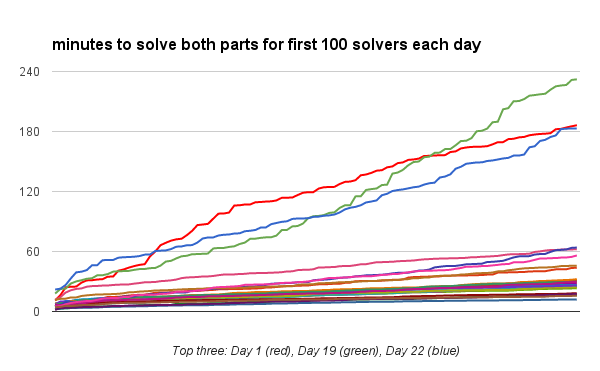The number of minutes it took the first 100 solvers each day to solve both parts of the puzzle. The three slowest days were Day 1 (red), which had a lot fewer users working on it at first than any other day, and Days 19 (green) and 22 (blue), which were two of the hardest puzzles.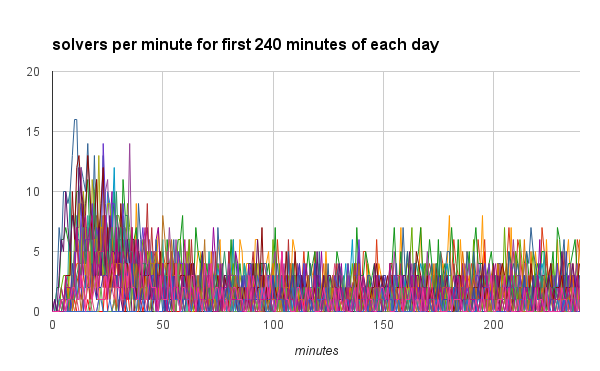The number of solvers each minute for the first 240 minutes after each puzzle unlocked. The days aren't labeled, because it's just a blob at this scale. Makes a nice curve, though; almost a Poisson distribution?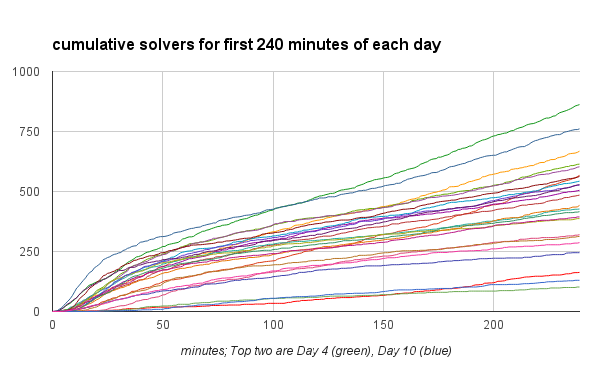The number of cumulative solvers for the first 240 minutes after each puzzle unlocked. The two days with the most solvers in that timespan were Days 4 (green) and 10 (blue).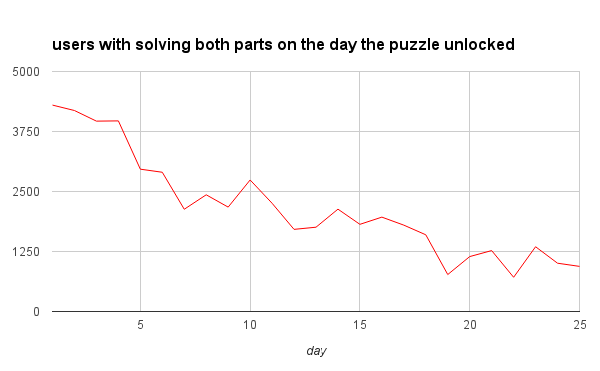The number of users that solved both parts of a puzzle within 24 hours of the puzzle unlocking. Even though Day 1 was a "slow" day in the graphs above, by the time the whole 24 hours is finished, Day 1 still comes out as the most-solved-on-that-day puzzle.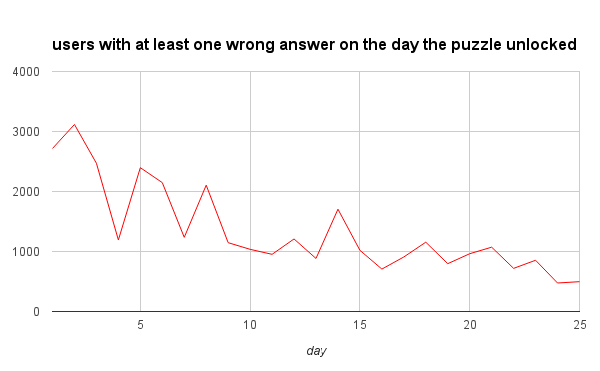The number of users with at least one wrong answer on a puzzle within 24 hours of the puzzle unlocking. Day 2 is the highest here, which I suspect is from the difficulty increasing to something that filtered out most of the non-programmers.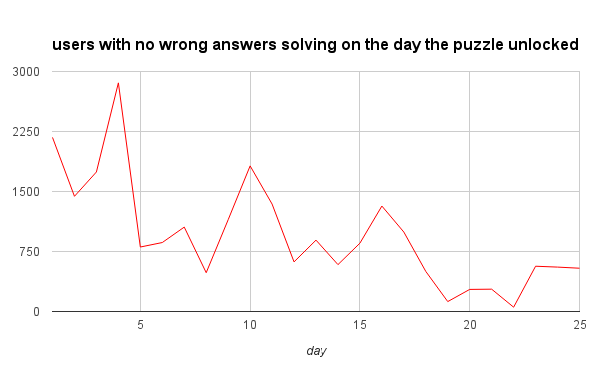The number of users that solved both parts of a puzzle with no wrong answers within 24 hours of the puzzle unlocking. This isn't quite the difference of the above two graphs, as the above "wrong answers" graph is all users, whereas this one only subtracts users with wrong answers that also solved both parts. The number of perfect solves on Days 19 and 22 was very low.